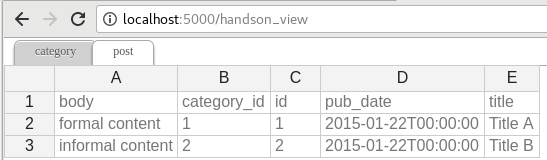C.W.

0.0.7

2021 年 07 月 10 日

```   用户: "我上传了一个 excel 文件但是你的网页说文件格式不支持。"

用户: "嗯，我不清楚。总之，我用的微软的 Excel 存的文件。哪一定是 excel 文件啦！"

"要么，将就一下。要么，把项目推迟 N 天。"
```

Flask-Excel 是基于 pyexcel 软件库。它的使命是让大家 在网站开发的时候，轻松的在数据存成 excel 文件让用户下载和处理用户上传的 excel 文件。 它可以把 excel 数据转换成二维数组，一维的字典数组和以excel 单页名为关键字，二维数组为值的字典；反之亦然。 这样的话，任由文件格式变化，你都可以在以下三个场景自由做数据转换:

1. excel 文件上传和下载

2. 数据库输入输出

3. excel 数据分析和存储

1. 不管是任何文件格式，函数界面不变

2. 不管是数据存在哪里，函数界面不变

1. 为 excel 数据导入数据库和从数据库输出数据为 excel 格式提供一站式服务

2. 把上传的 excel 文件直接转换成 Python 数据结构

3. 把 Python 数据结构转换为 excel 文件让用户下载

4. 在服务器里，把数据存成 excel 文件

5. 支持 csv, tsv, csvz, tsvz 格式。其他格式有以下软件包支持：

pyexcel-io

csv, csvz 1, tsv, tsvz 2

pyexcel-xls

pyexcel-xlsx

xlsx

openpyxl

pyexcel-ods3

ods

pyexcel-ezodf, lxml

pyexcel-ods

ods

odfpy

pyexcel-xlsxw

xlsx(write only)

XlsxWriter

pyexcel-libxlsxw

xlsx(write only)

libxlsxwriter

pyexcel-xlsxr

lxml

pyexcel-xlsbr

pyxlsb

pyexcel-odsr

lxml

pyexcel-odsw

write only for ods

loxun

pyexcel-htmlr

lxml,html5lib

pyexcel-pdfr

camelot

## 插件使用指南¶

Python 版本

pyexcel-text

write only:rst, mediawiki, html, latex, grid, pipe, orgtbl, plain simple read only: ndjson r/w: json

tabulate

2.6, 2.7, 3.3, 3.4 3.5, 3.6, pypy

pyexcel-handsontable

handsontable in html

handsontable

same as above

pyexcel-pygal

svg chart

pygal

2.7, 3.3, 3.4, 3.5 3.6, pypy

pyexcel-sortable

sortable table in html

csvtotable

same as above

pyexcel-gantt

gantt chart in html

frappe-gantt

except pypy, same as above

Footnotes

1

2

## 安装¶

```\$ pip install Flask-Excel
```

```\$ git clone https://github.com/pyexcel-webwares/Flask-Excel.git
\$ python setup.py install
```

```\$ pip install pyexcel-xlsx
```

## 配置¶

```import flask_excel as excel

...
excel.init_excel(app) # required since version 0.0.7
```

## 快速上手¶

``` 1# -*- coding: utf-8 -*-
2"""
3tiny_example.py
4:copyright: (c) 2015 by C. W.
6"""
9
11
12
15    if request.method == 'POST':
16        return jsonify({"result": request.get_array(field_name='file')})
17    return '''
18    <!doctype html>
20    <h1>Excel file upload (csv, tsv, csvz, tsvz only)</h1>
21    <form action="" method=post enctype=multipart/form-data><p>
22    <input type=file name=file><input type=submit value=Upload>
23    </form>
24    '''
25
26
29    return excel.make_response_from_array([[1, 2], [3, 4]], "csv")
30
31
32@app.route("/export", methods=['GET'])
33def export_records():
34    return excel.make_response_from_array([[1, 2], [3, 4]], "csv",
35                                          file_name="export_data")
36
37
40    return excel.make_response_from_array([[1, 2], [3, 4]], "csv",
41                                          file_name=u"中文文件名")
42
43
44# insert database related code here
45if __name__ == "__main__":
46    excel.init_excel(app)
47    app.run()
```

1. 一个用来展示 Excel 文件上传。

2. 三个用来展示 Excle 文件下载。

```<input ... name=file>
```

## 更多的文件格式¶

```pip install pyexcel-xls
pip install pyexcel-xlsx
pip install pyexcel-ods
```

## 数据库：数据输入和输出¶

# insert database related code here

```from flask_sqlalchemy import SQLAlchemy # sql operations
```

```pip install pyexcel-xls, pyexcel-handsontable
```

```app.config['SQLALCHEMY_DATABASE_URI'] = 'sqlite:///tmp.db'
db = SQLAlchemy(app)
```

```class Post(db.Model):
id = db.Column(db.Integer, primary_key=True)
title = db.Column(db.String(80))
body = db.Column(db.Text)
pub_date = db.Column(db.DateTime)

category_id = db.Column(db.Integer, db.ForeignKey('category.id'))
category = db.relationship('Category',
backref=db.backref('posts',
lazy='dynamic'))

def __init__(self, title, body, category, pub_date=None):
self.title = title
self.body = body
if pub_date is None:
pub_date = datetime.utcnow()
self.pub_date = pub_date
self.category = category

def __repr__(self):
return '<Post %r>' % self.title

class Category(db.Model):
id = db.Column(db.Integer, primary_key=True)
name = db.Column(db.String(50))

def __init__(self, name):
self.name = name

def __repr__(self):
return '<Category %r>' % self.name
```

```
db.create_all()

```

```@app.route("/import", methods=['GET', 'POST'])
def doimport():
if request.method == 'POST':

def category_init_func(row):
c = Category(row['name'])
c.id = row['id']
return c

def post_init_func(row):
c = Category.query.filter_by(name=row['category']).first()
p = Post(row['title'], row['body'], c, row['pub_date'])
return p
request.save_book_to_database(
field_name='file', session=db.session,
tables=[Category, Post],
initializers=[category_init_func, post_init_func])
return redirect(url_for('.handson_table'), code=302)
return '''
<!doctype html>
<form action="" method=post enctype=multipart/form-data><p>
</form>
'''
```

```@app.route("/export", methods=['GET'])
def doexport():
return excel.make_response_from_tables(db.session, [Category, Post], "xls")

``````@app.route("/handson_view", methods=['GET'])
def handson_table():
return excel.make_response_from_tables(
db.session, [Category, Post], 'handsontable.html')

```

## 输出过滤过的查询¶

```@app.route("/custom_export", methods=['GET'])
def docustomexport():
query_sets = Category.query.filter_by(id=1).all()
column_names = ['id', 'name']
return excel.make_response_from_query_sets(query_sets, column_names, "xls")

```

## 所有支持的数据结构¶

`get_dict()`

`make_response_from_dict()`

`get_records()`

`make_response_from_records()`

`get_array()`

`make_response_from_array()`

`get_book_dict()`

`make_response_from_book_dict()`

`pyexcel.Sheet`

`get_sheet()`

`make_response()`

`pyexcel.Book`

`get_book()`

`make_response()`

`make_response_from_a_table()`

`make_response_from_tables()`

`make_response_from_query_sets()`

`iget_records()`

`iget_array()`

## 支持¶## 函数参考¶

Flask-Excelpyexcel 函数安插到了 `flask.Request` 类里。

### ExcelRequest¶

• sheet_name – 对于多个表单的 excel 文件，它可以用来指定从哪一个表单取数据。缺省值是第一个表单。 要是 csv , tsv 文件的话，可以忽略 sheet_name

• keywords – 其他 `pyexcel.get_sheet()` 的参数

`pyexcel.Sheet`

```<!doctype html>
<h1>Excel file upload (csv, tsv, csvz, tsvz only)</h1>
<form action="" method=post enctype=multipart/form-data><p>
</form>
```

• field_name – 和前面 `get_sheet()` 一样。

• keywords – 其他 `pyexcel.get_book()` 的参数

`pyexcel.Book`

flask_excel.ExcelRequest.save_to_database(field_name=None, session=None, table=None, initializer=None, mapdict=None ** keywords)

flask_excel.ExcelRequest.isave_to_database(field_name=None, session=None, table=None, initializer=None, mapdict=None ** keywords)

`save_to_database()` 一样但用更少的内存。

flask_excel.ExcelRequest.save_book_to_database(field_name=None, session=None, tables=None, initializers=None, mapdicts=None, **keywords)

• field_name – 和前面 `get_sheet()` 一样。

• session – SQLAlchemy 的 session

• tables – 一组数据库表

• initializers – 一组自定义的初始化函数

• mapdicts – 一组表栏目适配字典。请注意，数据库表，初始化函数和栏目适配字典需要一一对应。

• keywords – 参照 `pyexcel.Book.save_to_database()`

flask_excel.ExcelRequest.isave_book_to_database(field_name=None, session=None, tables=None, initializers=None, mapdicts=None, **keywords)

`save_book_to_database()`. 一样但需要更少的内存

### Response methods¶

• pyexcel_instance`pyexcel.Sheet``pyexcel.Book`

• file_type

任何一个支持的文件类型，以下是可用的但不局限于它们的集合

• ’csv’

• ’tsv’

• ’csvz’

• ’tsvz’

• ’xls’

• ’xlsx’

• ’xlsm’

• ’ods’

• status – 允许开发人员发自定义的 http status

• file_name – 自定义的下载文件名称。注意，文件扩展名是不能改变的。